﻿ 自适应多阶段线性重构表示分类的人脸识别
«上一篇文章快速检索 高级检索

 智能系统学报2020, Vol. 15Issue (5): 964-971  DOI: 10.11992/tis.2019040020

### 引用本文QIAN Jianbin, CHEN Xiuhong. Self-adaptive multi-phase linear reconstruction representation based classification for face recognition[J]. CAAI Transactions on Intelligent Systems, 2020, 15(5): 964-971. DOI: 10.11992/tis.201904002.### 文章历史

Self-adaptive multi-phase linear reconstruction representation based classification for face recognition
QIAN Jianbin, CHEN Xiuhong
School of Digital Media, Jiangnan University, Wuxi 214122, China
Abstract: Representation-based classification (RBC) methods have recently been the promising pattern recognition technologies for object recognition. The representation coefficients of RBC as the linear reconstruction measure can be well used for classifying objects. But RBC methods performs very poorly on large-class-databases and in order to solve the problem of poor performance, a self-adaptive multi-phase linear reconstruction representation based classification (MPRBC) method is proposed. In this process, at first, the reconstruction coefficients regularized by L1-norm or L2-norm are obtained. Then the similar classes are selected according to the sum of the representation coefficients in each class, and all samples of similar classes are retained as training samples for the next stage. This strategy finally produces a sparse class probability distribution with higher classification confidence. The similar classes are selected adaptively according to the values of class coefficients, which improves the efficiency of the classification. Experimental results show that the proposed method is better than other RBC methods, especially on large-class-databases, and CPU time remains relatively low.
Key words: face recognition    self-adaptive    multi-phase    linear reconstruction    representation coefficient    classification method    sparse representation    collaborative representation    pattern recognition

1 相关RBC算法

1.1 基于线性重构度量的最近邻(LRMNN)分类

 $\mathop {{{\min}} }\limits_{{s}} \left\| {{{y}} - {{Xs}}} \right\|_2^2$ (1)

 $\mathop {{{\min}} }\limits_{{s}} \left\| {{{y}} - {{Xs}}} \right\|_2^2 + \lambda {\left\| {{s}} \right\|_p}$ (2)

 ${\hat{{s}}} = {({{{X}}^{\rm{T}}}{{X}} + {\rm{\lambda }}{{I}})^{ - 1}}{{{X}}^{\rm{T}}}{{y}}$ (3)

 $c = {\rm{arg ma}}{{\rm{x}}_{{{{c}}_i}}}\left\| {{{{\hat{{s}}}}_i}} \right\|$ (4)

1.2 表示系数之和(SoC)分类

SRC使用 ${L_1}$ 范数正则化约束，通过式(2)得到最优稀疏表示向量 ${\hat{{s}}}$ ，然后根据查询样本与训练样本的残差进行分类。SoC算法是在SRC的基础上用同类样本的表示系数之和对查询样本进行分类，其分类规则为

 $c = {\rm{arg ma}}{{\rm{x}}_{{{{c}}_i}}}\sum {{\delta ^{{{{c}}_i}}}} ({\hat{{s}}})$ (5)

1.3 两阶段线性重构度量分类(TPLRMC)

TPLRMC方法分为两个阶段。首先，利用正则化LRM模型获得查询样本的一个粗略的线性表示，并根据系数的大小依次从所有的训练样本中选择 $K$ 个近邻。假设对查询样本 ${{y}}$ 和训练样本集 ${{X}}$ ，由式(2)得到最优解为 ${\hat{{s}}}$ ，其分量是训练样本的表示系数。根据“训练样本的表示系数越大，则该训练样本对重构查询样本的贡献就越大”的原则[9,11]，将所有训练样本的表示系数按由大到小的顺序排序，并选出前 $K$ 个较大的系数所对应的样本，记为 ${\bar{{ X}}} = [{{\bar {{x}}}_1}\;\;{{\bar {{x}}}_2}\;\; \cdots \;\;{{\bar {{x}}}_K}]$ ，这些样本所在的类共H个，类集合记为 ${\bar {{C}}} = \{ {\bar c_1},\;{\bar c_2},\; \cdots ,\;{\bar c_H}\}$ ，它是所有样本类别的一个子集，即 ${\bar {{C}}} \subseteq \{ {c_1},\;{c_2},\; \cdots ,\;{c_M}\}$

 $\mathop {{{{\rm{min}}}} }\limits_{{s}} \left\| {{{y}} - {{{{\bar X}}s}}} \right\|_2^2 + \lambda {\left\| {{s}} \right\|_p}$ (6)

2 自适应多阶段线性重构表示分类

2.1 MPRBC的基本思想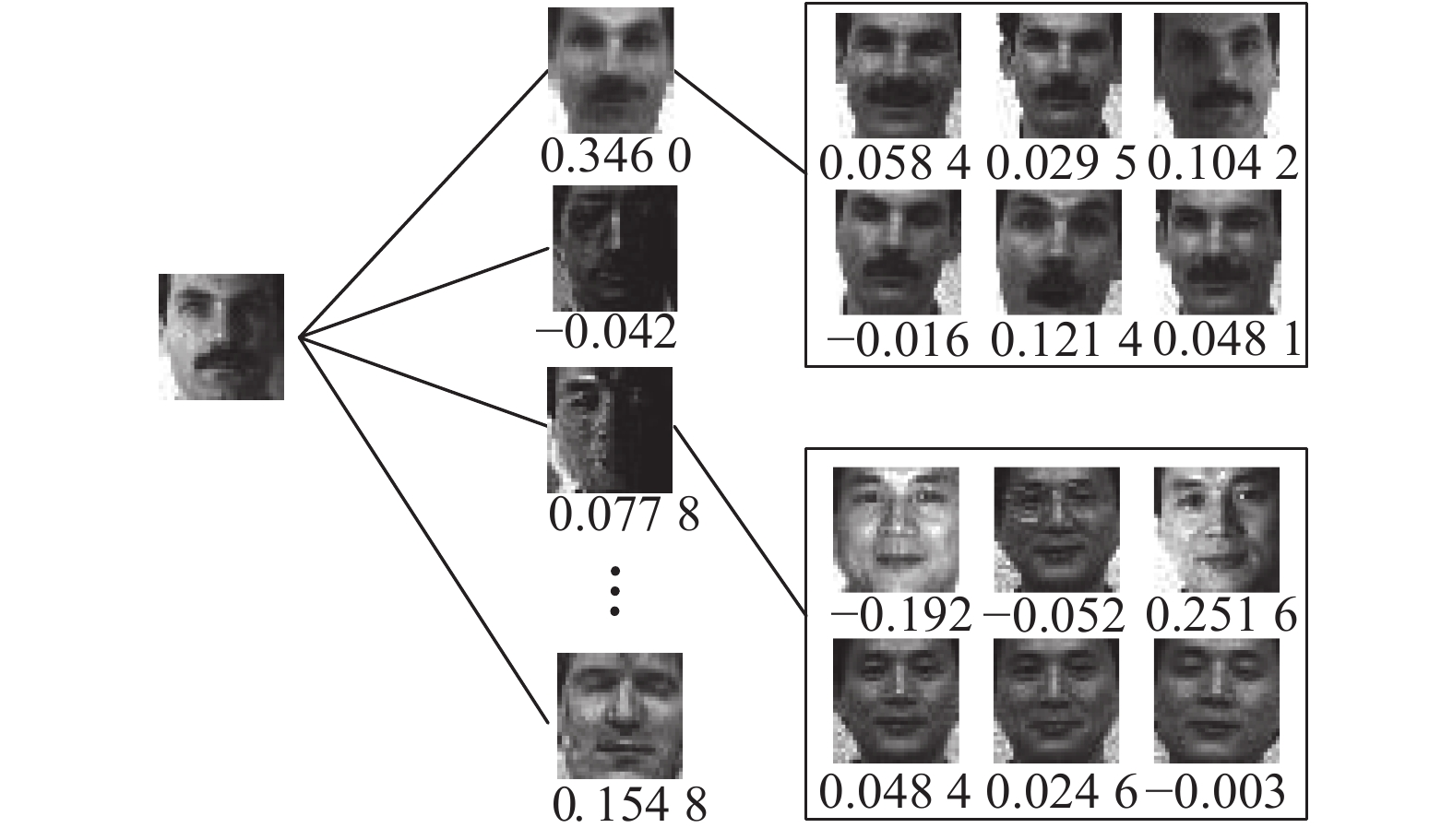Download: 图 1 部分训练样本表示系数 Fig. 1 Representation coefficients of some training samples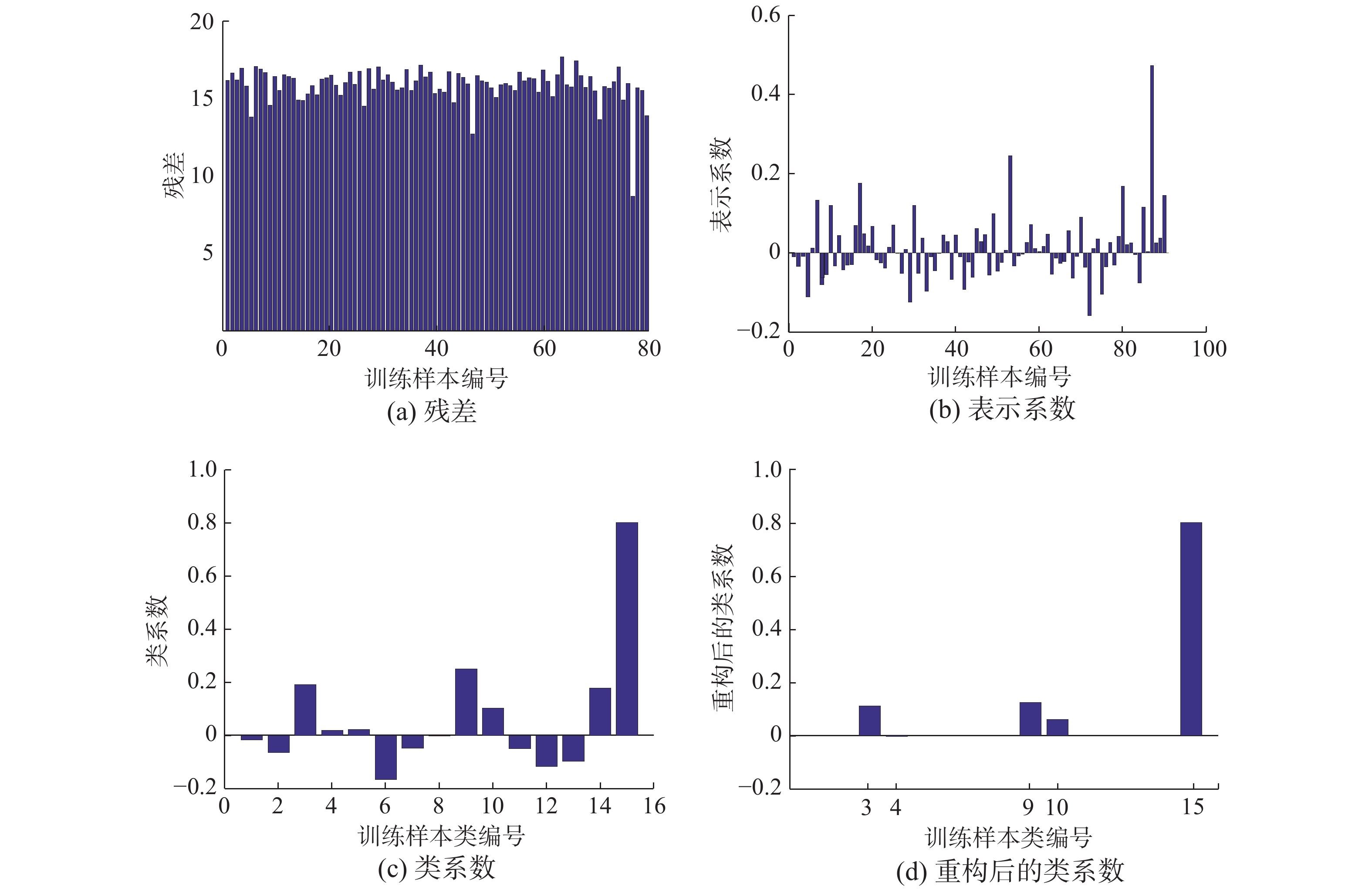Download: 图 2 不同算法的残差或(类)系数 Fig. 2 Residuals or (class) coefficients of different algorithms
2.2 MPRBC的实现过程

 $\mathop {{\rm{min}}}\limits_{{s}} \left\| {{{y}} - {{{X}}_k}{{s}}} \right\|_2^2 + {\rm{\lambda }}{\left\| {{s}} \right\|_p}$ (7)

 $c = {\rm{arg ma}}{{\rm{x}}_{{{{c}}_i}}}\sum {{\delta ^{{{{c}}_i}}}} ({{{s}}_k})$ (8)

2.3 自适应MPRBC算法

 ${M_k} = \mathop {\arg \max }\limits_{1 \leqslant M \leqslant {M_{k - 1}}} \left( {\sum_{i = 1}^M {{\rm{SU}}{{\rm{M}}^{{{{c}}_i}}} < } \alpha \times {\rm{SU}}{{\rm{M}}^{{{{c}}^ + }}}} \right)$ (9)

1) While ${M_k}$ 大于设定阈值 $\beta$ ，通过式(7)得出训练样本 ${{{X}}_k}$ 的表示系数 ${{{s}}_k}$

2) 根据式(9)确定 ${M_{k + 1}}$

3) 选取与前 ${M_{k + 1}}$ 个较大的 ${\rm{SU}}{{\rm{M}}^{{{{c}}_i}}}$ 所对应的类中所有样本，构成训练集 ${{{X}}_{k + 1}}$

4) $k = k + 1$

5) End while；

6) 根据式(8)确定查询样本 ${{y}}$ 的类别 ${{c}}$

3 实验结果及分析表 1 各人脸数据集的属性及特点 Tab.1 Attributes and characteristics of some face databases
3.1 识别精度的实验与分析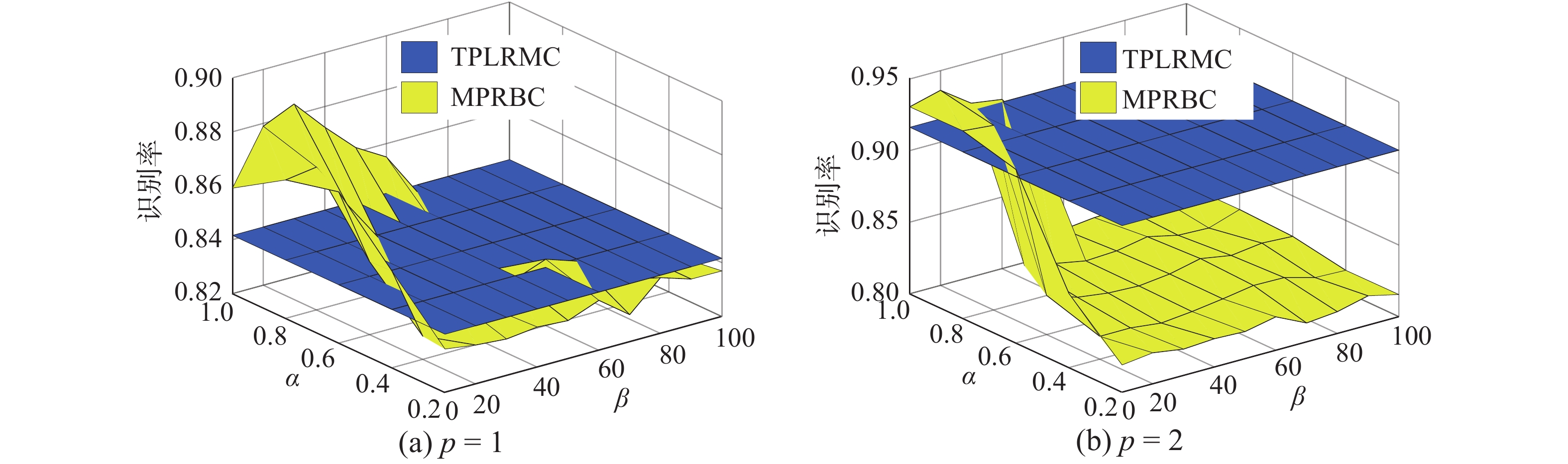Download: 图 3 AR人脸数据集上的识别率 Fig. 3 Recognition rates on AR face database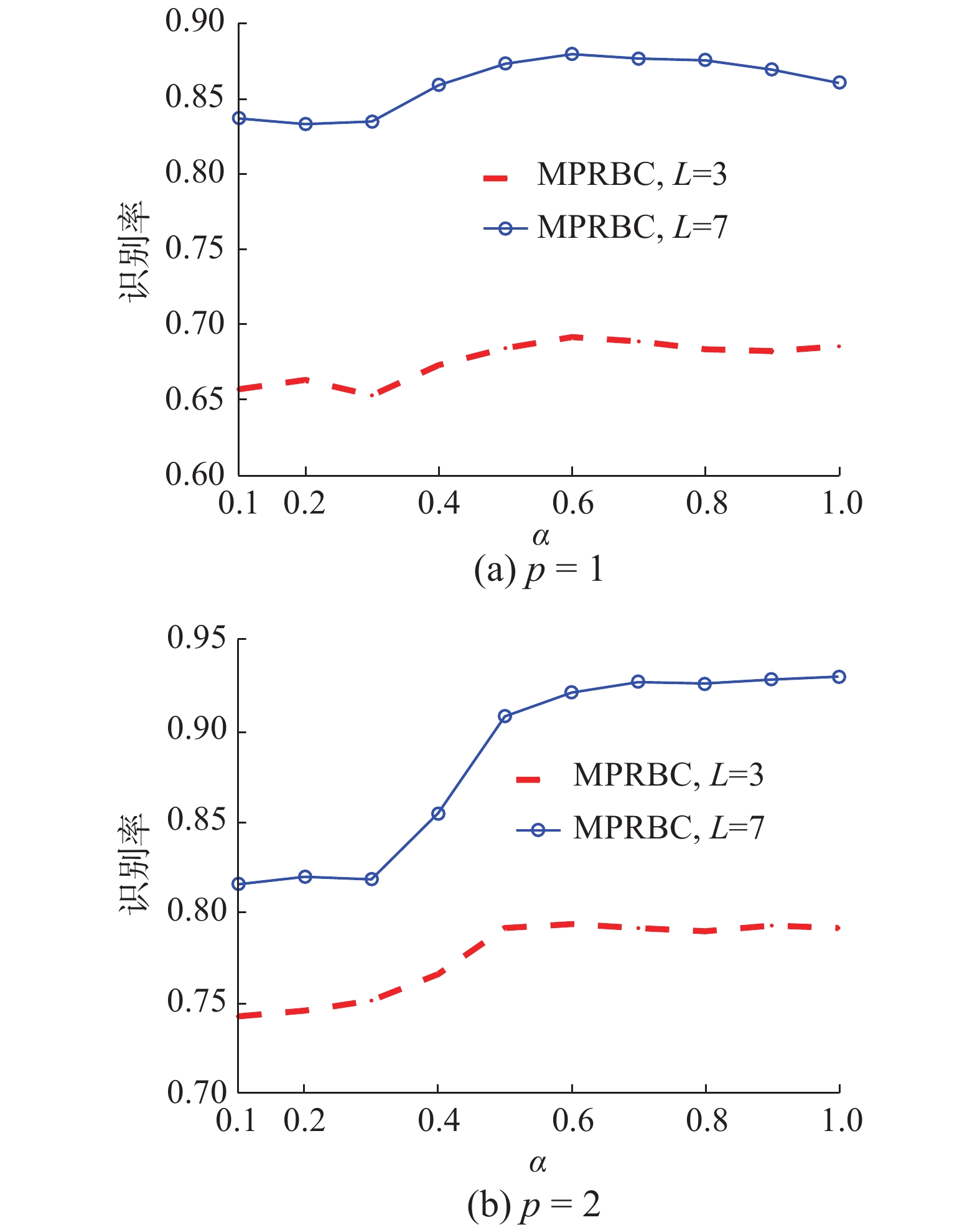Download: 图 4 参数α的影响 Fig. 4 Influence of parameter α表 2 各人脸数据集上的识别精度 Tab.2 Recognition accuracy of some face databases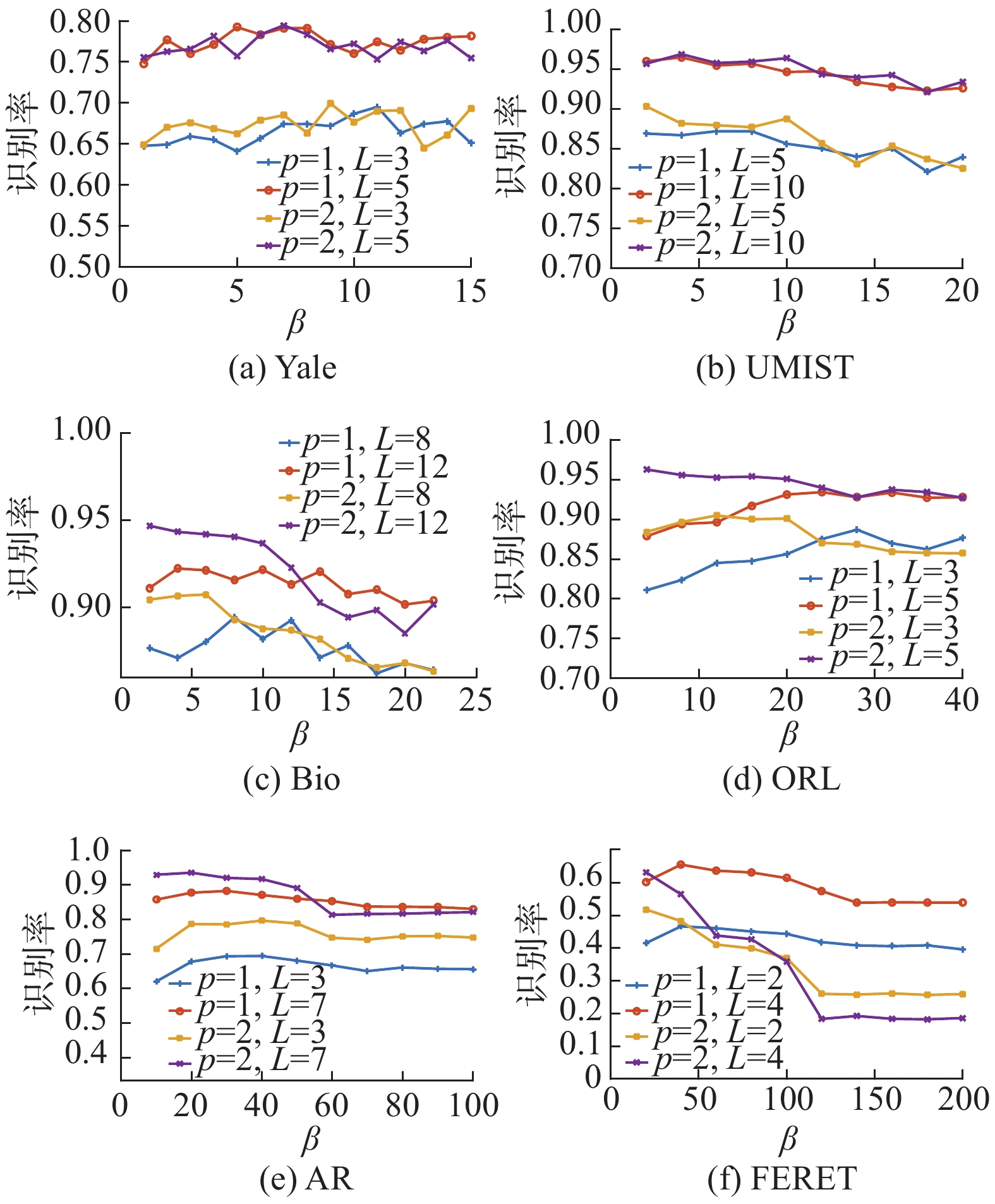Download: 图 5 MPRBC算法在各人脸数据集上的识别率 Fig. 5 Recognition rates of MPRBC on some face databases
3.2 时间复杂度的实验与分析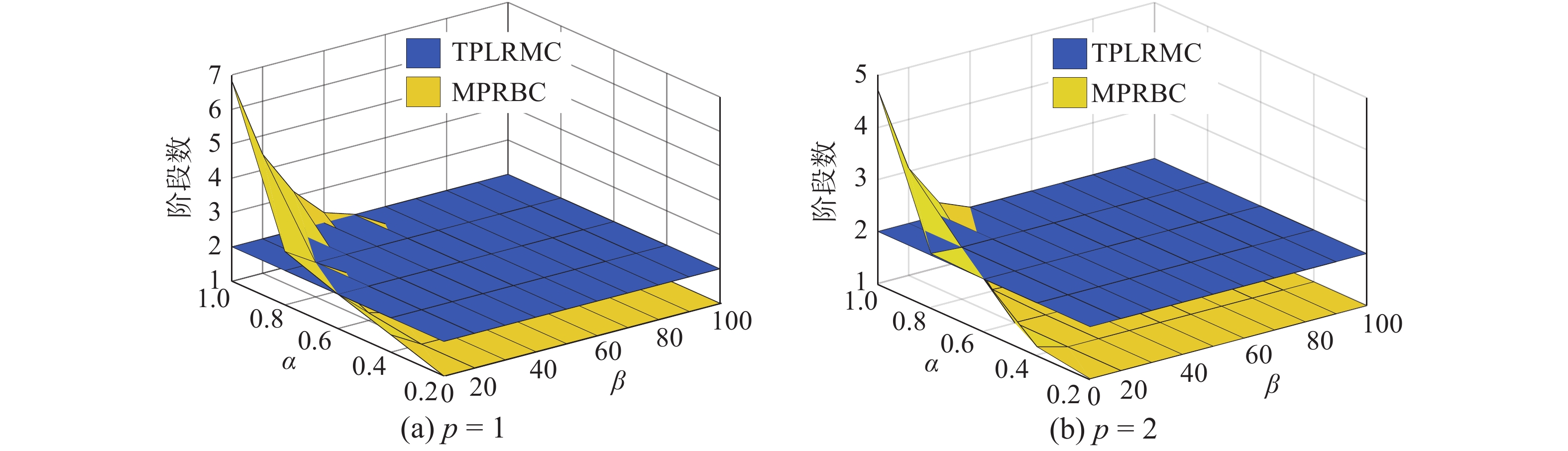Download: 图 6 MPRBC的阶段数 Fig. 6 Phase number of MPRBC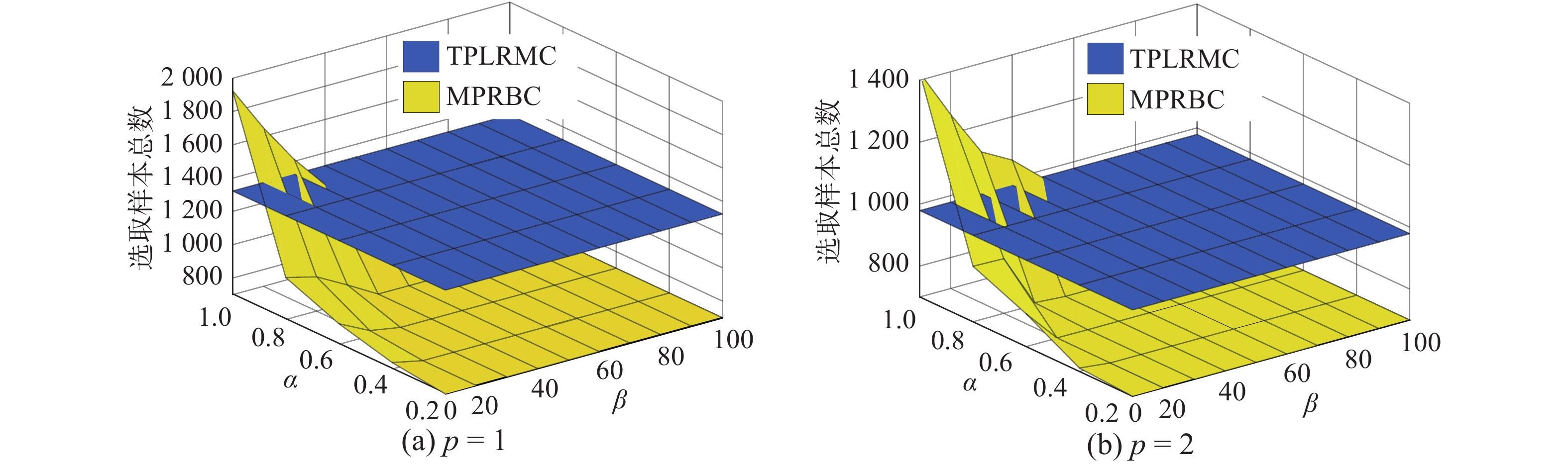Download: 图 7 MPRBC各阶段训练样本总数 Fig. 7 Total number of training samples of MPRBC at each stage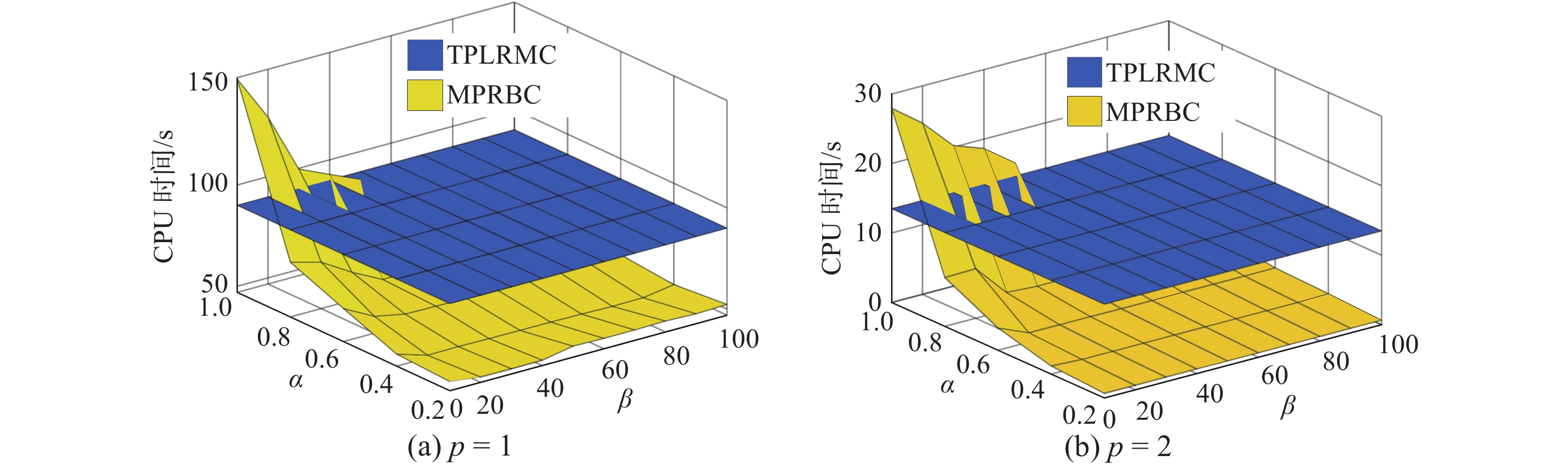Download: 图 8 MPRBC的CPU时间 Fig. 8 CPU times of MPRBC

$\alpha$ =1时，MPRBC算法在3个数据集上的最高识别精度所对应的CPU时间如表3所示，表中数据括号内为MPRBC算法所用平均阶段数。通过实验观察，在 ${L_1}$ 范数约束下，随着样本类别数的增加，MPRBC(p=1) 算法的阶段数也增加，算法的CPU时间也呈现递增趋势。在 ${L_2}$ 范数约束下，MPRBC(p=2)算法的CPU时间较高，这可能是因为不同类的训练样本在重构查询样本时其差异性不足，需要通过增加阶段数来提高识别精度。而由图3图8可知，减小 $\alpha$ 值，在识别性能优于TPLRMC的情况下，MPRBC仍能减小算法的CPU时间。总之，自适应算法在保证较高识别精度的同时，由于对各查询样本进行差异化地表示，算法的CPU时间保持相对较低水平。表 3 CPU时间 Tab.3 CPU times
4 结束语

  ZHANG Lei, YANG Meng, FENG Xiangchu. Sparse representation or collaborative representation: which helps face recognition[C]//Proceedings of the 2011 International Conference on Computer Vision. Barcelona, Spain, 2011: 471−478. (0)  XU Yong, LI Xuelong, YANG Jian, et al. Integrating conventional and inverse representation for face recognition[J]. IEEE transactions on cybernetics, 2014, 44(10): 1738-1746. DOI:10.1109/TCYB.2013.2293391 (0)  WRIGHT J, YANG A Y, GANESH A, et al. Robust face recognition via sparse representation[J]. IEEE transactions on pattern analysis and machine intelligence, 2009, 31(2): 210-227. DOI:10.1109/TPAMI.2008.79 (0)  XU Yong, ZHANG D, YANG Jian, et al. A two-phase test sample sparse representation method for use with face recognition[J]. IEEE transactions on circuits and systems for video technology, 2011, 21(9): 1255-1262. DOI:10.1109/TCSVT.2011.2138790 (0)  ZHANG Jian, YANG Jian. Linear reconstruction measure steered nearest neighbor classification framework[J]. Pattern recognition, 2014, 47(4): 1709-1720. DOI:10.1016/j.patcog.2013.10.018 (0)  CHENG H, LIU Zicheng, HOU Lei, et al. Sparsity-induced similarity measure and its applications[J]. IEEE transactions on circuits and systems for video technology, 2016, 26(4): 613-626. DOI:10.1109/TCSVT.2012.2225911 (0)  LI Jiang, LU Canyi. A new decision rule for sparse representation based classification for face recognition[J]. Neurocomputing, 2013, 116: 265-271. DOI:10.1016/j.neucom.2012.04.034 (0)  GAO Shenghua, TSANG I W H, CHIA L T. Laplacian sparse coding, hypergraph laplacian sparse coding, and applications[J]. IEEE transactions on pattern analysis and machine intelligence, 2013, 35(1): 92-104. DOI:10.1109/TPAMI.2012.63 (0)  QIAO Lishan, CHEN Songcan, TAN Xiaoyang. Sparsity preserving projections with applications to face recognition[J]. Pattern recognition, 2010, 43(1): 331-341. DOI:10.1016/j.patcog.2009.05.005 (0)  MA Hongxing, GOU Jianping, WANG Xili, et al. Sparse coefficient-based k-nearest neighbor classification[J]. IEEE access, 2017, 5: 16618-16634. DOI:10.1109/ACCESS.2017.2739807 (0)  GOU Jianping, XU Yong, ZHANG D, et al. Two-phase linear reconstruction measure-based classification for face recognition[J]. Information sciences, 2018, 433–434: 17-36. DOI:10.1016/j.ins.2017.12.025 (0)  YAN Shuicheng, XU Dong, ZHANG Benyu, et al. Graph embedding and extensions: a general framework for dimensionality reduction[J]. IEEE transactions on pattern analysis and machine intelligence, 2007, 29(1): 40-51. DOI:10.1109/TPAMI.2007.250598 (0)  FANG Xiaozhao, XU Yong, LI Xuelong, et al. Learning a nonnegative sparse graph for linear regression[J]. IEEE transactions on image processing, 2015, 24(9): 2760-2771. DOI:10.1109/TIP.2015.2425545 (0)  叶志飞, 文益民, 吕宝粮. 不平衡分类问题研究综述[J]. 智能系统学报, 2009, 4(2): 148-156. YE Zhifei, WEN Yimin, LV Baoliang. A survey of imbalanced pattern classification problems[J]. CAAI transactions on intelligent systems, 2009, 4(2): 148-156. (0)  LIU Fan, TANG Jinhui, SONG Yan, et al. Local structure based multi-phase collaborative representation for face recognition with single sample per person[J]. Information sciences, 2016, 346–347: 198-215. DOI:10.1016/j.ins.2016.02.001 (0)  QIAN J B. data[EB/OL]. Github, 2019 (2019−05−30) [2019−05]. https://github.com/greatQntgz/MPRBC (0)